NEET  >  DC Pandey Solutions: Laws of Motion - 1

# DC Pandey Solutions: Laws of Motion - 1 - Notes | Study Physics Class 11 - NEET

 Table of contentsIntroductory Exercise 5.11 Crore+ students have signed up on EduRev. Have you?

Introductory Exercise 5.1

Q.1. The diagram shows a rough plank resting on a cylinder with one end of the plank on rough ground. Neglect friction between plank and cylinder. Draw diagrams to show:
(a) the forces acting on the plank,
(b) the forces acting on the cylinder.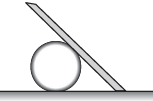Sol.

N = Normal force on cylinder by plank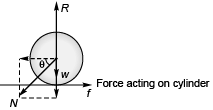R = Normal force on cylinder by ground,
f = Force of friction by ground by cylinder,
w = Weight of cylinder.
N cosθ = f
w + N sinθ = R,
N(R) = Reaction to N,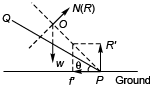(Force acting on plank)
i.e., normal force on plank by cylinder
R' = Normal force on plank by ground,
w = Weight of plank,
f' = frictional force on plank by ground.
Resultant of f' and R', N(R) and w pass through point O.

Q.2. Two spheres A and B are placed between two vertical walls as shown in figure. Friction is absent everywhere. Draw the free body diagrams of both the spheres.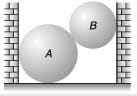Sol.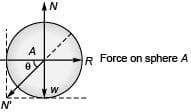R = Normal force on sphere A by left wall,
N = Normal force on sphere A by ground,
N' = Normal force on sphere A by sphere B,
wA = Weight of sphere A.
N' cos θ = R
N' sin θ + wA = N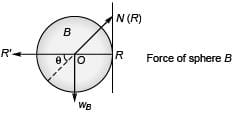R' = Normal force on sphere B by right wall,
N ( R) = Reaction to N i.e. normal force on sphere B by sphere A,
wB = Weight of sphere B,
R', N(R) and wB pass through point O, the centre sphere B.

Q.3. A point A on a sphere of weight W rests in contact with a smooth vertical wall and is supported by a string joining a point B on the sphere to a point C on the wall. Draw free body diagram of the sphere.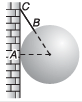Sol:
N = Normal force on sphere by wall,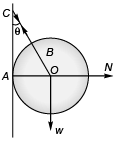w = Weight of sphere,
T = Tension in string.

Q.4. Write down the components of four forces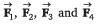along ox and oy directions as shown in Fig. 5.33.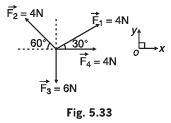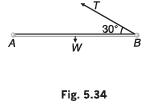Sol.

1. F1x = 2√3 N
2. F2x = -2 N
3. F3x = 0
4. F4x = 4 N
5. F1y = 2N
6. F2y = 2√3 N
7. F3y = -6 N
8. F4y = 0

Component of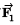along x-axis : 4 cos 30° = 2√3 N
along y-axis : 4 sin 30° = 2 N
Component of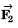along x-axis : 4 cos 120° = - 2 N
along y-axis : 4 sin 120° = 2√3 N
Component of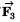along x-axis : 6 cos 270° = 0 N
along y-axis : 6 sin 270° = - 6 N
Component of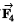along x-axis : 4 cos 0° = 4 N
along y-axis : 4 sin 0° = 0 N

Q.5. A uniform rod AB of weight w is hinged to a fixed point at A It is held in the horizontal position by a string, one end of which is attached to B as shown in Fig. 5.34. Find in terms of w, the tension in the string.

Sol: Taking moment about point A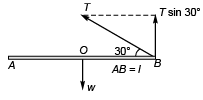AB = 1
(T sin 30) 1 = w 1/2
⇒ T = w

Q.6. In Question 3 of the same exercise, the radius of the sphere is a. The length of the string is also a. Find the tension in the string.

Sol.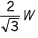See figure (answer to question no. 3)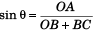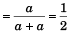T cos 30° = w
or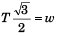or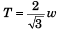Q.7. Find the values of the unknown forces if the given set of forces shown in figure below are in equilibrium.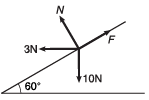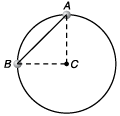Sol. F = 10.16 newton, R = 2.4 newton

R cos 30° + 3 = f cos 60°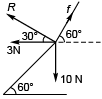i.e.,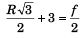or
R√3 + 6 = f …(i)
and R sin 30° + f sin 60° = 10
i.e.,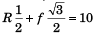or R + f√3 = 20 …(ii)
Substituting the value of f from Eq. (i) in Eq. (ii)
R + ( R√3 + 6)√3 = 20
4 R + 6√3 = 20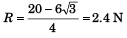∴   f = (2.4) √3 + 6
f = 10.16 N

Q.8. Two beads of equal masses m are attached by a string of length √2a and are free to move in a smooth circular ring lying in a vertical plane as shown in Fig. 5.36. Here, a is the radius of the ring. Find the tension and acceleration of B just after the beads are released to move.

Sol.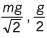At point B (instantaneous vertical acceleration only)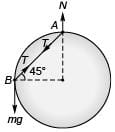∴  mg - T sin 45° = ma …(i)
At point A (instantaneous horizontal acceleration only)
∴  T cos 45° = ma …(ii)
Combining Eqs. (i) and (ii)
mg - ma = ma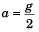T cos 45° = m(g/2)

T/√2 = m(g/2)

T = mg/√2

The document DC Pandey Solutions: Laws of Motion - 1 - Notes | Study Physics Class 11 - NEET is a part of the NEET Course Physics Class 11.
All you need of NEET at this link: NEET

## Physics Class 11

127 videos|464 docs|210 tests

## Physics Class 11

127 videos|464 docs|210 tests

### How to Prepare for NEET

Read our guide to prepare for NEET which is created by Toppers & the best Teachers

Track your progress, build streaks, highlight & save important lessons and more!

,

,

,

,

,

,

,

,

,

,

,

,

,

,

,

,

,

,

,

,

,

;